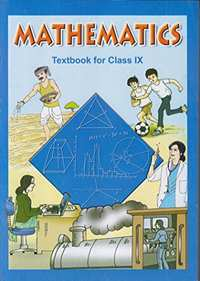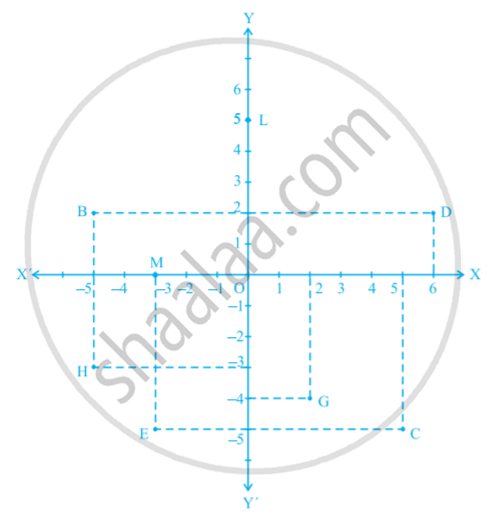# NCERT solutions for Class 9 Maths chapter 3 - Coordinate Geometry [Latest edition]

#### Chapters## Solutions for Chapter 3: Coordinate Geometry

Below listed, you can find solutions for Chapter 3 of CBSE NCERT for Class 9 Maths.

Exercise 3.1Exercise 3.2Exercise 3.3
Exercise 3.1 [Page 53]

### NCERT solutions for Class 9 Maths Chapter 3 Coordinate Geometry Exercise 3.1 [Page 53]

Exercise 3.1 | Q 1 | Page 53

How will you describe the position of a table lamp on your study table to another person?

Exercise 3.1 | Q 2 | Page 53

(Street Plan) : A city has two main roads which cross each other at the centre of the city. These two roads are along the North-South direction and East-West direction.

All the other streets of the city run parallel to these roads and are 200 m apart. There are 5 streets in each direction. Using 1cm = 200 m, draw a model of the city on your notebook. Represent the roads/streets by single lines.

There are many cross- streets in your model. A particular cross-street is made by two streets, one running in the North - South direction and another in the East - West direction. Each cross street is referred to in the following manner : If the 2nd street running in the North - South direction and 5th in the East - West direction meet at some crossing, then we will call this cross-street (2, 5). Using this convention, find:-

(i) how many cross - streets can be referred to as (4, 3).

(ii) how many cross - streets can be referred to as (3, 4).

Exercise 3.2 [Page 60]

### NCERT solutions for Class 9 Maths Chapter 3 Coordinate Geometry Exercise 3.2 [Page 60]

Exercise 3.2 | Q 1.1 | Page 60

Write the answer of the following question:-

What is the name of horizontal and the vertical lines drawn to determine the position of any point in the Cartesian plane?

Exercise 3.2 | Q 1.2 | Page 60

Write the answer of each of the following questions:-

What is the name of each part of the plane formed by these two lines?

Exercise 3.2 | Q 1.3 | Page 60

Write the answer of each of the following questions:-

Write the name of the point where these two lines intersect.

Exercise 3.2 | Q 2. (i) | Page 60

See the given figure, and write the following:-

The coordinates of B.Exercise 3.2 | Q 2. (ii) | Page 60

See the given figure, and write the following:

The coordinates of C.Exercise 3.2 | Q 2. (iii) | Page 60

See the given figure, and write the following:

The point identified by the coordinates (–3, –5)Exercise 3.2 | Q 2. (iv) | Page 60

See the given figure, and write the following:

The point identified by the coordinates (2, – 4)Exercise 3.2 | Q 2. (v) | Page 60

See the given figure, and write the following:

The abscissa of the point DExercise 3.2 | Q 2. (vi) | Page 60

See the given figure, and write the following:

The ordinate of the point H.Exercise 3.2 | Q 2. (vii) | Page 60

See the given figure, and write the following:

The coordinates of the point L.Exercise 3.2 | Q 2. (viii) | Page 60

See the given figure, and write the following:

The coordinates of the point M.Exercise 3.3 [Page 65]

### NCERT solutions for Class 9 Maths Chapter 3 Coordinate Geometry Exercise 3.3 [Page 65]

Exercise 3.3 | Q 1 | Page 65

In which quadrant or on which axis do each of the points (– 2, 4), (3, – 1), (– 1, 0), (1, 2) and (– 3, – 5) lie? Verify your answer by locating them on the Cartesian plane.

Exercise 3.3 | Q 2 | Page 65

Plot the points (x, y) given in the following table on the plane, choosing suitable units of distance on the axes.

 x -2 -1 0 1 3 y 8 7 -1.25 3 -1

## Solutions for Chapter 3: Coordinate Geometry

Exercise 3.1Exercise 3.2Exercise 3.3## NCERT solutions for Class 9 Maths chapter 3 - Coordinate Geometry

Shaalaa.com has the CBSE Mathematics Class 9 Maths CBSE solutions in a manner that help students grasp basic concepts better and faster. The detailed, step-by-step solutions will help you understand the concepts better and clarify any confusion. NCERT solutions for Mathematics Class 9 Maths CBSE 3 (Coordinate Geometry) include all questions with answers and detailed explanations. This will clear students' doubts about questions and improve their application skills while preparing for board exams.

Further, we at Shaalaa.com provide such solutions so students can prepare for written exams. NCERT textbook solutions can be a core help for self-study and provide excellent self-help guidance for students.

Concepts covered in Class 9 Maths chapter 3 Coordinate Geometry are Cartesian Coordinate System, Plotting a Point in the Plane If Its Coordinates Are Given., Coordinate Geometry.

Using NCERT Class 9 Maths solutions Coordinate Geometry exercise by students is an easy way to prepare for the exams, as they involve solutions arranged chapter-wise and also page-wise. The questions involved in NCERT Solutions are essential questions that can be asked in the final exam. Maximum CBSE Class 9 Maths students prefer NCERT Textbook Solutions to score more in exams.

Get the free view of Chapter 3, Coordinate Geometry Class 9 Maths additional questions for Mathematics Class 9 Maths CBSE, and you can use Shaalaa.com to keep it handy for your exam preparation.

Share